# Clinical Trials Programming Using SAS 9 v6.0

Page:    1 / 7
Exam contains 108 questions

Given the following entry in the SAS log:
Which alternate method could you use to?
Transpose all data
Not produce a warning message in the log

• A. Use PROC TRANSPOSE with a WHERE clause to omit records with missing VISIT values
• B. Use PROC COPY with SELECT statements
• C. Use PROC DATASETS with SELECT and MODIFY statements
• D. Use a DATA step with conditional OUTPUT statements

Given the following partial output data set: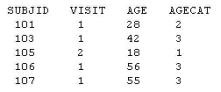Which code was used to create AGECAT?

• A. if age <18 then AGECAT=1; if 18<=AGE<=40 then AGECAT=2; else AGECAT=3;
• B. if age <=18 then do AGECAT=1; else if 18<AGE<=40 then do AGECAT=2; else do AGECAT=3;
• C. if age <18 then AGECAT=1; else if 18<=AGE<=40 then AGECAT=2; else AGECAT=3;
• D. if age <=18 then AGECAT=1; else if 18<AGE<=40 then AGECAT=2; else AGECAT=3;

The following SAS program is submitted:
Which types of variables are DayofMonth, MonthofYear, and Year?

• A. DayofMonth, Year, and MonthofYear are character.
• B. DayofMonth, Year, and MonthofYear are numeric.
• C. DayofMonth and Year are numeric. MonthofYear is character
• D. DayofMonth, Year, and MonthofYear are date values

Given the following data set (AE):
Data will be reported by onset week. Day 1 ?7 is Week 1, Day 8 ?14 is Week 2. Events beyond Day 14 are assigned Week 3 and will be reported as Follow-up events.
Which statements properly assign WEEK to each event?

• A. if day > 14 then week = 3 ; else if day > 7 then week = 2 ; else if day > 0 then week = 1 ;
• B. if day > 0 then week = 1 ; else if day > 7 then week = 2 ; else if day > 14 then week = 3 ;
• C. select ; when (day > 0) week = 1 ; when (day > 7) week = 2 ; otherwise week = 3 ; end ;
• D. select ; when (day > 14) week = 3 ; when (day > 7) week = 2 ; otherwise week = 1 ; end ;

Study day is defined as DCMDATE minus RFSTDTC +1
Which statement will compute the study day correctly without producing notes for missing values in the log?

• A. STUDYDAY=DCMDATE-RFSTDTC+1;
• B. STUDYDAY=input(DCMDATE,yymmdd8.)-input(RFSTDTC,date9.)+1;
• C. If RFSTDTC^='' and length(DCMDATE)=8 then STUDYDAY=input(DCMDATE,yymmdd8.)-input(RFSTDTC,date9.)+1;
• D. If RFSTDTC^='' and length(DCMDATE)=8 then STUDYDAY=input(DCMDATE,date9.)- input(RFSTDTC,yymmdd8.)+1;

A Treatment-Emergent Adverse Event (TEAE) is commonly defined as any event that occurs on or after the date and time of:

• A. informed consent
• B. baseline assessment
• C. study enrollment
• D. first dose of study drug

The following question will ask you to provide a line of missing code.
Given the following data set work.vs:
The following SAS program is submitted to create a new data set that carries forward the previous value of sbp when the value is missing.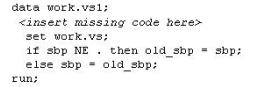In the space below, enter the line of code that completes the program (Case is ignored. Do not add leading or trailing spaces to your answer.).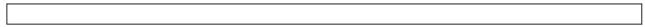This question will ask you to provide a section of missing code.
Given the input SAS data set LABRAW:
Which DO LOOP will create the output SAS data set WORK.LAB_NEW?

• A. do i=1 to 2; visit=i; date=dat{i}; result=num{i}; output; end;
• B. do i=1 to 2; visit=i; date=dat{i}; result=num{i}; end; output;
• C. do i=1 to 2; do j=1 to 2; visit=i; date=dat{j}; result=num{j}; output; end;
• D. do i=1 to 2; do j=1 to 2; visit=i; date=dat{j}; result=num{j}; end; output; end;

Given the following SCORE data set:
Based on the concept of Last Observation Carried Forward, what will be the value for

SCORE for the Week 12 -

• A. 157
• B. 152
• C. missing
• D. 151

The following SAS program is submitted:
What is the value of the variable day when the data step completes?

• A. 1
• B. 6
• C. 7
• D. 8

Given the SAS data set containing subject's phone numbers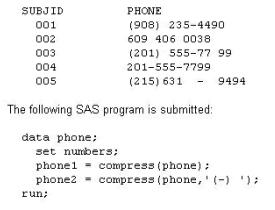What is the value of PHONE2 for subject 005?

• A. (215) 631-9494
• B. 2156319494
• C. (215) 6319494
• D. 631-9494

Given the following vital signs data:
Baseline is defined as the last non-missing value prior to Day 1.
What is the value for the change from baseline for Week 1 for Subject 2?

• A. -23
• B. 11
• C. -11
• D. 23

This question will ask you to provide a line of missing code.
Given the following data set LABS(only first 10 lines shown):
In the space below, enter the statement that completes the program to produce the desired output (Case is ignored. Do not add leading or trailing spaces to your answer.).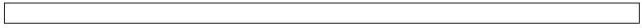Given the following data set WORK.DM:
Which subjects will appear in the LOG file?

• A. 001 and 004
• B. 001 and 006
• C. 003 and 004
• D. 004 and 006

This question will ask you to provide lines of missing code.
Given the following SCORE data set:
Variable LOCF contains the imputed score that would replace the missing SCORE value
(based on last observation carried forward method). Which SAS statements complete the program?

• A. LOCF = lag(score) ; if first.subject then LOCF = . ; if score ^= . then LOCF = score ;
• B. if first.subject then LOCF = . ; if score = . then LOCF = lag(score) ;
• C. retain LOCF ; if first.subject then LOCF = . ; if score ^= . then LOCF = score ;
• D. retain score ; if first.subject then LOCF = . ; if score ^= . then LOCF = score ;